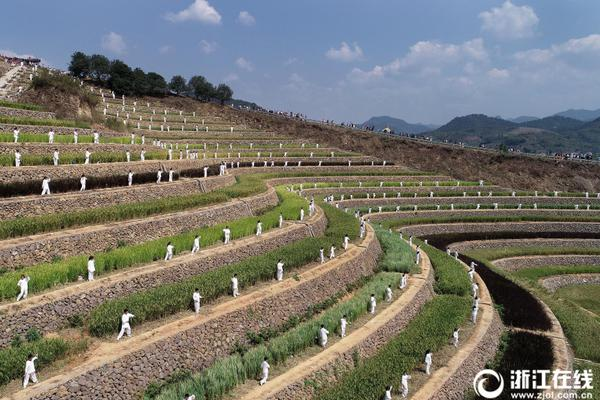## 焦点图片

32支龙舟队6日金山湖竞渡

“法治在线”第52期聚焦夫妻共同财产

“好人微访谈”对话博罗县博仁文化志愿者协会会长吴炳菊

3岁男童被卡悬空热心群众及时救下

“嫁给”深山17年守护茂林情不变

functioncreatePageHTML(_nPageCount,_nCurrIndex,_sPageName,_sPageExt){ if(_nPageCount==null){ varprevPage=_nCurrIndex-1//上一页 varnextPage=_nCurrIndex+1//下一页 if(_nPageCount>1&&_nCurrIndex!=0&&_nCurrIndex!=1){ document.write("
• <<
• <
• "); elseif(_nPageCount>1&&_nCurrIndex!=0&&_nCurrIndex==1){ document.write("
• <<
• <
• "); document.write("
• <<
• <
• "); varnum=20;//每组显示页码数量 for(vari=0+(_nCurrIndex-_nCurrIndex%num);i<=(num-1+(_nCurrIndex-_nCurrIndex%num))&&(i<_nPageCount);i++){ document.write(""+(i+1)+""); document.write("
• "+(i+1)+"
• "); document.write("
• "+(i+1)+"
• "); if(_nPageCount>1&&_nCurrIndex!=(_nPageCount-1)){ document.write("
• ><\/a><\/li>
• >>
• "); document.write("
• >
• >>
• ");

金山湖公园二期或春节前开放

周末去大亚湾海滨体验渔家风情

游走博罗古村落探寻生态之美短视频|第一身份

标签：